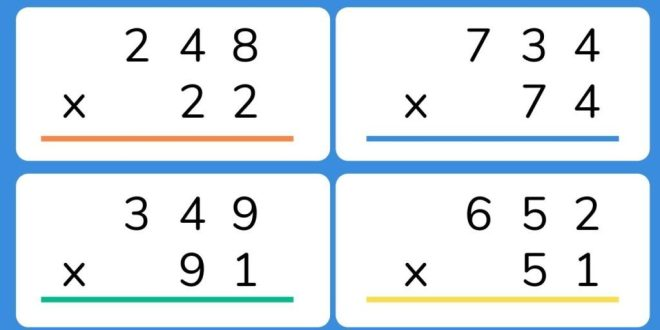Breaking News# Multiplication Chart 1-20 : Unlocking the Power of Multiplication

In the realm of mathematics, multiplication is a fundamental operation that plays a crucial role in various mathematical concepts and real-life applications. To aid in the understanding and mastery of multiplication, educators and mathematicians have developed tools and resources, such as the multiplication chart. This article aims to provide a comprehensive analysis of the multiplication chart 1-20, exploring its structure, benefits, and practical applications.

## Understanding the Multiplication Chart

The multiplication chart, also known as a times table or multiplication table, is a visual representation of the multiplication facts from 1 to 20. It consists of a grid with rows and columns, where each cell represents the product of the corresponding row and column numbers. For instance, the cell at row 3 and column 4 would display the product of 3 multiplied by 4, which is 12.

The chart is organized in a systematic manner, with numbers increasing from left to right and top to bottom. The rows represent the multiplicand, while the columns represent the multiplier. This arrangement allows for quick reference and easy identification of multiplication facts.

## Benefits of Using the Multiplication Chart

The multiplication chart serves as a valuable tool for both students and educators. Here are some key benefits of using the multiplication chart:

1. Visual Representation: The chart provides a visual representation of multiplication facts, making it easier for students to grasp the concept and identify patterns. By observing the chart, students can recognize symmetries, such as the commutative property (e.g., 3 x 4 = 4 x 3) and patterns in products (e.g., multiples of 10).

2. Memorization Aid: Memorizing multiplication facts can be challenging for many students. The multiplication chart serves as a memory aid, allowing students to quickly recall multiplication facts by referring to the chart. As students repeatedly use the chart, they gradually internalize the multiplication facts, leading to improved fluency and efficiency in mental calculations.

3. Multiplication Strategies: The chart can be used as a tool to develop multiplication strategies. For example, students can use the chart to identify patterns in products and derive shortcuts, such as multiplying by 10 or using doubling and halving techniques. These strategies not only enhance mental math skills but also foster a deeper understanding of multiplication concepts.

4. Multiplication Fact Practice: The multiplication chart can be utilized for practice and reinforcement of multiplication facts. Students can engage in various activities, such as filling in missing products, identifying factors, or solving puzzles based on the chart. This interactive approach promotes active learning and helps students consolidate their knowledge of multiplication.

## Practical Applications of the Multiplication Chart

The multiplication chart extends beyond the realm of the classroom and finds practical applications in everyday life. Here are a few examples:

1. Mathematical Problem Solving: The chart is a valuable tool for solving mathematical problems that involve multiplication. Whether it’s calculating the total cost of multiple items or determining the dimensions of a rectangular area, the chart provides a quick reference for finding products and solving equations.

2. Time Management: The multiplication chart is useful for time management tasks that involve scheduling and calculating durations. For instance, when planning a timetable or organizing events, the chart can assist in determining the duration of each activity by multiplying time slots with the number of repetitions.

3. Scaling and Proportions: In various fields, such as architecture, engineering, and design, scaling and proportions play a vital role. The multiplication chart aids in scaling objects or drawings by multiplying dimensions with a common factor. It enables professionals to maintain accurate proportions while resizing or enlarging objects.

## Enhancing Multiplication Skills Beyond the Chart

While the multiplication chart is an invaluable resource, it is essential to develop a deeper understanding of multiplication concepts. Here are some strategies to enhance multiplication skills beyond the chart:

1. Conceptual Understanding: Encourage students to explore the underlying concepts of multiplication, such as repeated addition, equal groups, and the distributive property. By understanding the principles behind multiplication, students can apply their knowledge to solve complex problems.

2. Real-Life Applications: Integrate real-life applications of multiplication into the curriculum. This can include tasks like calculating grocery bills, determining distances, or analyzing data sets. By connecting multiplication to everyday situations, students develop a practical understanding of its significance.

3. Mental Math Practice: Encourage mental math exercises to improve fluency and efficiency in multiplication. Engage students in activities that require quick mental calculations, such as mental multiplication races or timed quizzes. These exercises strengthen mental math skills and reduce reliance on external aids like the multiplication chart.

## Conclusion:

The multiplication chart 1-20 serves as a powerful tool for understanding and mastering multiplication. Its systematic structure, visual representation, and numerous benefits make it an indispensable resource for students and educators alike. By utilizing the chart effectively and complementing it with a deeper conceptual understanding, students can unlock the full potential of multiplication and apply it to various real-life scenarios.